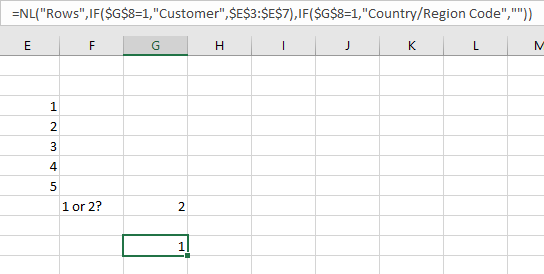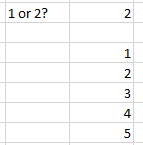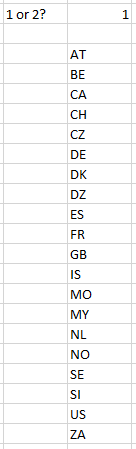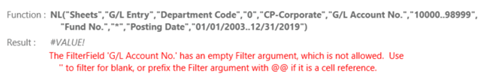I'd like to be able to create sheets either based on the Dimension one (Department Code), or on a preset range on the options page.   When I entered this formula with the If function and two NL Sheets functions, Excel faults and closes.  Is there a work around to be able to accomplish the same?

=IF(\$D\$2=2,NL("Sheets","G/L Entry","Department Code","0",\$L\$19,"Department Code",Dept,"G/L Account No.",\$L\$8,"Fund No.","*","Posting Date",\$L\$5),NL("Sheets",Options!\$D\$121:\$D\$122))

•Harry Lewis

Hi Bob -

The Jet replicating functions [ NL(Rows), NL(Columns), and NL(Sheets) ] cannot be enclosed within an Excel IF() function.

You would need to use Conditional Replication... by placing your IF() within the NL(Sheets) function.

•Bob Thorp

•Bob Thorp

I modified the formula, but Excel does not accept it as written. =NL("Sheets",if(\$D\$2=2,("G/L Entry","Department Code","0",\$L\$19,"Department Code",Dept,"G/L Account No.",\$L\$8,"Fund No.","*","Posting Date",\$L\$5),(Options!\$D\$121:\$D\$122)))

I'm not sure what is incorrect since Excel shows the formula as =NL("Sheets",if(\$D\$2=2,(TRUE),(FALSE)))

What do I have worng?

•Harry Lewis

Hi Bob -

Here is a simplified version of what you are attempting.

Here's my report where by both my table name and field are "conditionalized" (ya gotta love making up words):When I run my report withe a value of 2, I get the list from the specified range:When run with a value of 1, I get the desired field:Your situation is obviously more complex, and you would have to conditionalize the filters, as well.

•Bob Thorp

Hi Harry,  I think I have followed your format, but Excel does not accept the formula.  It appears that I just don't have my parenthesis in the correct places, but grouping look correct. Can you tell what I have wrong?  =NL("Sheets",if(\$D\$2=2,("G/L Entry","Department Code","0",\$L\$19,"Department Code",Dept,"G/L Account No.",\$L\$8,"Fund No.","*","Posting Date",\$L\$5),if(\$D\$2=1,Options!\$D\$121:\$D\$122),""))

•Harry Lewis

Hi Bob -

Each parameter of the NL(Sheets) function would need to have an IF() function included:

`=NL("Sheets",IF(\$D\$2=2,"table name",range),IF(\$D\$2=2,"field name",""),IF(\$D\$2=2,"filter field",""),IF(\$D\$2=2,"filter","")...`

So the first part of your function would look like:

=NL("Sheets",if(\$D\$2=2,"G/L Entry",Options!\$D\$121:\$D\$122),IF(\$D\$2=2,"Department Code",""),IF(\$D\$2=2","Company=
,""),IF(\$D\$2=2,\$L\$19,""),IF(\$D\$2=2,"Department Code",""),IF(\$D\$2=2,Dept,"")...

Think of it this way...

The NL function has these parameters:

NL(  WHAT, TABLE, FIELD, FilterField1, Filter1, FilterField2, Filter2, etc.)

You function needs to say:

• if my condition is met, the TABLE is X, otherwise the table is Y
• if my condition is met, the FIELD is a, otherwise the FIELD is b
• if my condition is met, FilterField1 is c, otherwise FilterField1 is d
• if my condition is met, Filter1 is e, otherwise Filter1 is f
• etc, etc, etc.

•Bob Thorp

Hi Harry,  I have enter the formula and it is accepted but renders a blank.  When I use the JET Function wizard to evaluate the formula it shows the Filter Field "G/L Account No. has an empty filter argument which is not allowed.  I'm confused as it shows the G/L account range, 10000..98999, in the formula.  So, now what do I have wrong?  Hey, I really appreciate your help with this as it has not been intuitive to figure out.

=NL("Sheets",IF(\$D\$2=2,"G/L Entry",Options!\$D\$121:\$D\$122),IF(\$D\$2=2,"Department Code",""),IF(\$D\$2=2,"0",""),IF(\$D\$2=2,\$L\$19,""),IF(\$D\$2=2,"G/L Account No.",""),IF(\$D\$2=2,\$L\$8,""),IF(\$D\$2=2,"Fund No.",""),IF(\$D\$2=2,"*",""),IF(\$D\$2=2,"Posting Date",""),IF(\$D\$2=2,\$L\$5,""))•Harry Lewis

Hi Bob -

What version of Jet Reports are you running?

(by the way, if you are always going to use * for filtering your Fund, there's not reason to filter it)

Edited by Harry Lewis
•Bob Thorp

JET Professional 2018 18.1.18149.1 on our server which has more horsepower than my laptop which is running

JET Report 2019 19.3.18339.1.

•Harry Lewis

Let's run a quick test before I aim you to Jet Tech Support...

What happens if you try to enter your function in Excel on your laptop?

•Bob Thorp

Sorry for the delay, but I was working from home and wanted to confirm this is still an issue when I'm in the office with the best connection to our server.  The formula shown below still returned a blank. After removing the filters for G/L Account No., Fund and Posting Date, the formula still returned a blank. I added a filter for Department <>’’ and that portion of the formula is now working as expected!!!

=NL("Sheets",IF(\$D\$2=2,"G/L Entry",Options!\$D\$121:\$D\$122),IF(\$D\$2=2,"Department Code",""),IF(\$D\$2=2,"0",""),IF(\$D\$2=2,\$L\$19,""),IF(\$D\$2=2,"G/L Account No.",""),IF(\$D\$2=2,\$L\$8,""),IF(\$D\$2=2,"Fund No.",""),IF(\$D\$2=2,"*",""),IF(\$D\$2=2,"Posting Date",""),IF(\$D\$2=2,\$L\$5,""))

Now, I'd like to make the Options!\$D\$121:\$D\$122 option dynamic.  I'll submit a new post for it.

Edited by Bob Thorp